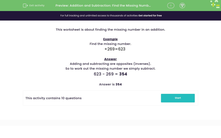# Find the Missing Number by Using Inverse Operation

In this worksheet, students will subtract to find the missing number in an addition.Key stage:  KS 2

Curriculum topic:   Number: Addition and Subtraction

Curriculum subtopic:   Add/Subtract up to Four-Digit Numbers

Difficulty level:#### Worksheet Overview

Example

Find the missing number.

+ 269 = 623

Adding and subtracting are opposites (inverses).

So, to work out the missing number we simply subtract.

623 - 269 = 354

Are you ready to have a go at some questions now?### What is EdPlace?

We're your National Curriculum aligned online education content provider helping each child succeed in English, maths and science from year 1 to GCSE. With an EdPlace account you’ll be able to track and measure progress, helping each child achieve their best. We build confidence and attainment by personalising each child’s learning at a level that suits them.

Get started